Error Correcting Codes, Perfect Hashing Circuits, and Deterministic Dynamic Dictionaries Peter Bro Miltersen June 1997

### Abstract:

We consider dictionaries of size n over the finite universe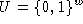and introduce a new technique for their implementation: error correcting codes. The use of such codes makes it possible to replace the use of strong forms of hashing, such as universal hashing, with much weaker forms, such as clustering.

We use our approach to construct, for any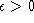, a deterministic solution to the dynamic dictionary problem using linear space, with worst case time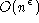for insertions and deletions, and worst case time O(1) for lookups. This is the first deterministic solution to the dynamic dictionary problem with linear space, constant query time, and non-trivial update time. In particular, we get a solution to the static dictionary problem with O(n) space, worst case query time O(1), and deterministic initialization time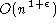. The best previous deterministic initialization time for such dictionaries, due to Andersson, is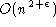. The model of computation for these bounds is a unit cost RAM with word size w (i.e. matching the universe), and a standard instruction set. The constants in the big-O's are independent upon w. The solutions are weakly non-uniform in w, i.e. the code of the algorithm contains word sized constants, depending on w, which must be computed at compile-time, rather than at run-time, for the stated run-time bounds to hold.

An ingredient of our proofs, which may be interesting in its own right, is the following observation: A good error correcting code for a bit vector fitting into a word can be computed in O(1) time on a RAM with unit cost multiplication.

As another application of our technique in a different model of computation, we give a new construction of perfect hashing circuits, improving a construction by Goldreich and Wigderson. In particular, we show that for any setof size n, there is a Boolean circuit C of size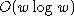with w inputs and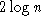outputs so that the function defined by C is 1-1 on S. The best previous bound on the size of such a circuit was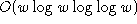Available as PostScript, PDF, DVI.